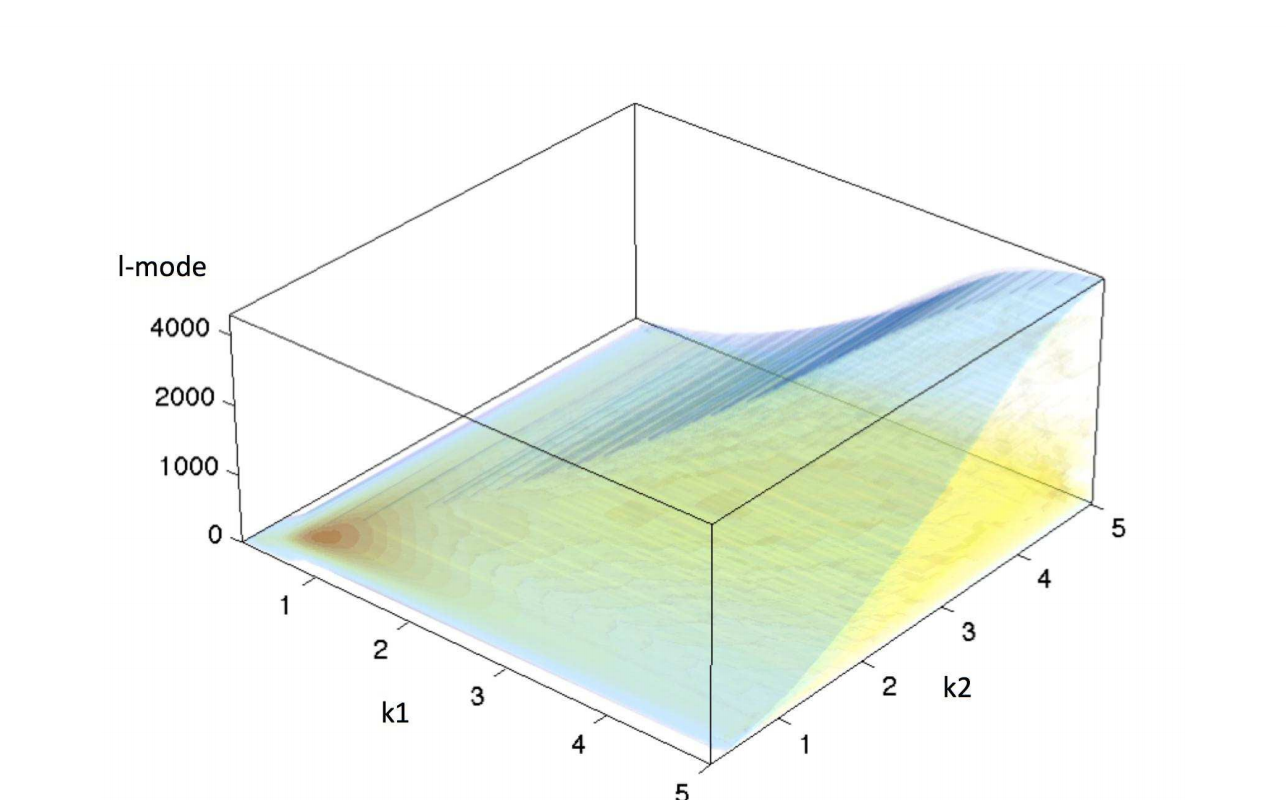# 3D Cosmic Shear: Cosmology from CFHTLenSAuthors: T. D. Kitching, ... , M. Kilbinger, et al. Journal: MNRAS Year: 2014 Download: ADS | arXiv

## Abstract

This paper presents the first application of 3D cosmic shear to a wide-field weak lensing survey. 3D cosmic shear is a technique that analyses weak lensing in three dimensions using a spherical harmonic approach, and does not bin data in the redshift direction. This is applied to CFHTLenS, a 154 square degree imaging survey with a median redshift of 0.7 and an effective number density of 11 galaxies per square arcminute usable for weak lensing. To account for survey masks we apply a 3D pseudo-Cl approach on weak lensing data, and to avoid uncertainties in the highly non-linear regime, we separately analyse radial wave numbers k<=1.5h/Mpc and k<=5.0h/Mpc, and angular wavenumbers l~400-5000. We show how one can recover 2D and tomographic power spectra from the full 3D cosmic shear power spectra and present a measurement of the 2D cosmic shear power spectrum, and measurements of a set of 2-bin and 6-bin cosmic shear tomographic power spectra; in doing so we find that using the 3D power in the calculation of such 2D and tomographic power spectra from data naturally accounts for a minimum scale in the matter power spectrum. We use 3D cosmic shear to constrain cosmologies with parameters OmegaM, OmegaB, sigma8, h, ns, w0, wa. For a non-evolving dark energy equation of state, and assuming a flat cosmology, lensing combined with WMAP7 results in h=0.78+/-0.12, OmegaM=0.252+/-0.079, sigma8=0.88+/-0.23 and w=-1.16+/-0.38 using only scales k<=1.5h/Mpc. We also present results of lensing combined with first year Planck results, where we find no tension with the results from this analysis, but we also find no significant improvement over the Planck results alone. We find evidence of a suppression of power compared to LCDM on small scales 1.5 < k < 5.0 h/Mpc in the lensing data, which is consistent with predictions of the effect of baryonic feedback on the matter power spectrum.